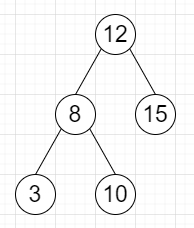# Program to find sum each of the diagonal path elements in a binary tree in Python

Suppose we have a binary tree, we have to find the sum of each of the diagonals in the tree starting from the top to bottom right.

So, if the input is likethen the output will be [27, 18, 3] as the diagonals are [12,15], [8,10],. So the sum values are [27, 18, 3]

To solve this, we will follow these steps −

Define a function traverse() . This will take node, numLeft, output

• if node is null, then

• return

• if numLeft >= size of output , then

• insert data of node at the end of output

• otherwise,

• output[numLeft] := output[numLeft] + data of node

• if left of node is not null, then

• traverse(left of node, numLeft+1, output)

• if right of node is not null, then

• traverse(right of node, numLeft, output)

• From the main method, do the following −

• output := a new list

• traverse(root, 0, output)

• return output

Let us see the following implementation to get better understanding −

## Example

Live Demo

class TreeNode:
def __init__(self, data, left = None, right = None):
self.data = data
self.left = left
self.right = right
class Solution:
def solve(self, root):
output = []
def traverse(node, numLeft, output):
if not node:
return
if numLeft >= len(output):
output.append(node.data)
else:
output[numLeft] += node.data
if node.left:
traverse(node.left, numLeft+1, output)
if node.right:
traverse(node.right, numLeft, output)
traverse(root, 0, output)
return output
ob = Solution()
root = TreeNode(12)
root.left = TreeNode(8)
root.right = TreeNode(15)
root.left.left = TreeNode(3)
root.left.right = TreeNode(10)
print(ob.solve(root))

## Input

root = TreeNode(12)
root.left = TreeNode(8)
root.right = TreeNode(15)
root.left.left = TreeNode(3)
root.left.right = TreeNode(10)

## Output

[27, 18, 3]